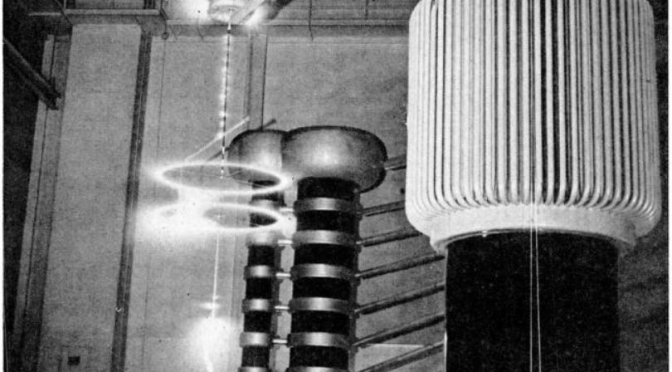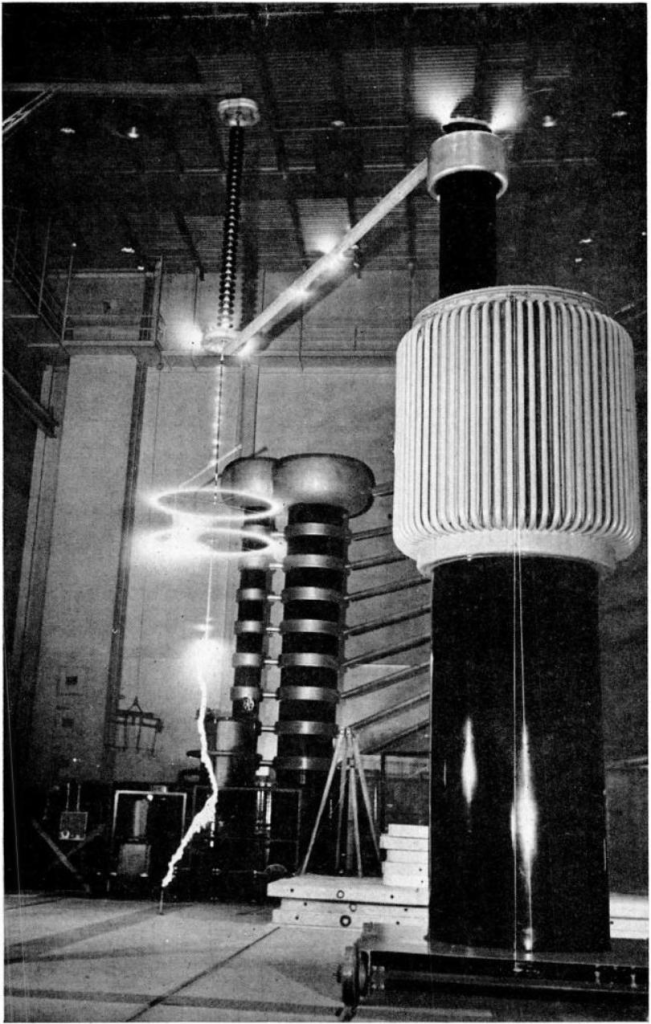# The Fallacy of Conductors

Article by Eric Dollard, Wireless Engineer (1987).

### 1.

When electro-magnetic energy is conveyed from one point in space to another point in space a closed loop is required to connect the point of generation with the point of utilization. This closed loop is called the electric circuit and consists of a boundary formed by what have become known as electric conductors. This boundary encloses a definite quantity of space.

When electro-magnetic energy flows through the space enclosed by the electric circuit phenomena take place inside the circuit material as well as the space outside this material.

Within the circuit conductor material, during the passage of electro-magnetic energy, this energy is continuously being consumed within the molecular space and converted into thermo-dynamic energy (heat). This may be represented by the passing electro-magnetic wave dragging into the electric circuit material. This drag is analogous to frictional losses and is called the resistance of the electric circuit, R.

In the space outside the circuit conductor material, during the passage of electro-magnetic energy, a condition of aetheric stress exists, which is called the electric field of the electric circuit. The energy contained by the electric field is continuously being transferred through this space from the point of generation which supplies energy to the electric field to the point of utilization which abstracts energy from the electric field.

The electric field of the circuit exerts physical magnetic and dielectric actions. The magnetic action is orientated parallel to the surface of the conductor material (in its immediate vicinity). That is, a needle shaped magnetic body tends to set itself in a direction parallel to the surface of the conductor material.

The dielectric action is orientated perpendicular to the surface of the conductor material (in its immediate vicinity). That is, a needle shaped dielectric body tends to set itself in a direction perpendicular to the surface of the conductor material. Thus, the electric field of the circuit, over which passes the flow of electro-magnetic energy, has three fundamental axes which are at right angles with each other:

The dielectric axis, perpendicular to the conductor surface,

The magnetic axis, parallel to the conductor surface,

The electro-magnetic axis, co-axial with the direction of the electric circuit.

The space outside of the conductor material, bounded by the electric circuit, has the property of propagating a wavefront of light at a definite velocity, C. This velocity is a characteristic property of the aether in which the electric circuit exists. The inverse square of this velocity is called the capacitance of the electric circuit.

```	C = 1/c2	(4 10-9 pi-1) Farads
```

The capacitance is a measure of the ability to store energy in the dielectric field of induction, of the electric circuit.

The quantity of space enclosed by the bounding electric circuit is proportional to the total length of the electric circuit, l1, multiplied by the distance between the bounding conductors, l2,

```	l1 l2 = l02	(centimetre)2
```

and has the dimensions of an area. This area in square centimetres defines what is called the inductance of the electric circuit.

```	l02 = L		4 10-9 pi Henrys
```

The inductance is a measure of the ability to store energy in the magnetic field of induction of the electric circuit.

Together, the capacitance and the inductance representing the dielectric and magnetic fields of induction of the electric circuit, serve as a measure of the propagation characteristics of the electric circuit for the transmission of electro-magnetic energy.

```	- LC  = t02	: natural period
- L/C = Z02	: natural impedance
```

### 2.

The popular conception of electro-magnetic energy transmission as it exists today is: energy is transmitted through the interior of the conductor material; that is, electricity flows through wires like water flows through pipes. This transmission is said to involve the flow of charged sub-atomic particles called electrons.

According to this theory the materials possessing the most “free electrons” serve as the best conductors of electro-magnetic energy. Conversely, the materials possessing the least “free electrons” serve as the poorest conductors of electro-magnetic energy. These materials are called insulators. Insulators are said to block the passage of electricity.

The conclusion drawn is that electricity is the flow of electrons and that the space outside of the conductor material is empty and dead. It follows that a superconductor is that material which offers no opposition to the flow of electrons and hence no opposition to the flow of electricity. Conversely, free space devoid of matter offers total opposition to the flow of electricity. Nothing could be further from the truth, yet this is the concept of electricity propounded by the scientist of today.

The real actions of the conducting material presents itself when it is in the so-called superconducting state. If a section of a superconducting material is suspended in space, free to move, and a magnetic field of induction is made to approach this material, it is found that the material is repelled by the approach of the field. If the material is indeed superconducting it will maintain a definite distance, l, for an indefinite period of time t -> infinity, from the source of magnetic induction. Any tendency for the material to sink into the magnetic field, l -> 0, indicates the material is not perfectly superconducting but has a finite resistance R.

It may be concluded that the so-called conducting material does not so much conduct as it does repel or reflect magnetism, or electro-magnetic energy in general.

If an electric circuit is conveying electro-magnetic energy as previously discussed it is found that a force or pressure is exerted upon the circuit material. This pressure tends to repel opposing parts of the circuit material and cause the circuit to expand. The quantity of this pressure in the space bounded by the circuit is called the magneto-motive force of the circuit.

It can therefore be seen that the conducting materials serve as the walls of a container holding magnetic pressure. If the conducting material is in the so-called superconducting state and the ends of the circuit are shorted the electric circuit will hold this magneto-motive pressure indefinitely, in analogy with compressed air stored in a tank. In order for this to be the result of electron flow requires that this flow be in perpetual motion, an unlikely proposition.

It may be concluded that materials called electric conductors might best be called electric obstructors and serve not to conduct electro-magnetism but serve to reflect it back on itself. The flow of electro-magnetism is conducted by the aethereous space bound by the obstructing material.

The character of this aethereous space is represented by its inductance L and its capacitance C. Since pure space is considered a perfect insulator by atomic theory, is it not ironic that it offers the least resistance to the flow of electro-magnetism? It is then the insulators that are the true conductors of electricity.

## The Eric Dollard Papers

Save nearly 25% when you buy the complete Eric Dollard Papers!

### INCLUDES:

Price: \$74.95 + shipping